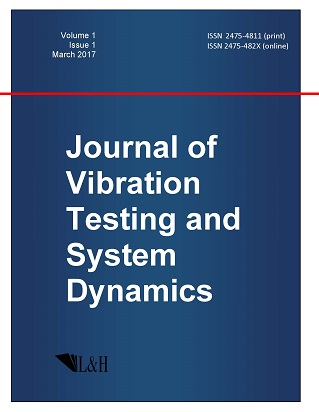ISSN: 2475-4811 (print)
ISSN: 2475-482X (online)
Journal of Vibration Testing and System Dynamics

C. Steve Suh (editor), Pawel Olejnik (editor),

Xianguo Tuo (editor)

Pawel Olejnik (editor)

Lodz University of Technology, Poland

Email: pawel.olejnik@p.lodz.pl

C. Steve Suh (editor)

Texas A&M University, USA

Email: ssuh@tamu.edu

Xiangguo Tuo (editor)

Sichuan University of Science and Engineering, China

Email: tuoxianguo@suse.edu.cn

Symbolic Methods for Estimating the Sets of Solutions of Ordinary Differential Equations with Perturbations on a Finite Time Interval

Journal of Vibration Testing and System Dynamics 7(1) (2023) 31--37 | DOI:10.5890/JVTSD.2023.03.005

A. N. Rogalev

Institute of Computational Modelling SB RAS, Krasnoyarsk, 660036, Russia

Abstract

In many papers and reports of academician N.N. Yanenko, problem statements are presented that require computing the accuracy of numerical results, depending on perturbations. Such problems are equivalent to problems of stability research. The problems of motion stability over a finite time interval are of considerable interest. This is also due to the fact that most of the methods for studying stability determine stability as $t \to \infty$. Practical stability over a finite time interval means that the solutions are uniformly bounded with respect to the set of initial values and the totality of disturbing influences.

References

1.   Martynyuk, A.A. (2007), Stability of a set of trajectories of nonlinear dynamics, Doklady Akademii Nauk, 414(3), 299-303 (in Russian).
2.   Novikov, V.A. and Rogalev, A.N. (1990), The influence of the wrapping effect on finding upper and lower estimates of solutions of systems of ordinary differential equations, USSR Computational Mathematics and Mathematical Physics, 29(10), 229-231.
3.   Novikov, V.A. and Rogalev, A.N. (1993), The construction of convergent upper and lower bounds of the solution of systems of ordinary differential equations with initial interval data, Computational Mathematics and Mathematical Physics, 33(2), 193-203.
4.   Rogalev, A.N. (1995), Finding optimal guaranteed estimates of solution sets for ODE systems with interval data, Computing Technologies, 4(13), 58-64 (in Russian).
5.   Rogalev, A.N. (2003), Inclusion of sets of solutions of differential equations and guaranteed global error estimates, Computing Technologies, 8(6), 80-94 (in Russian).
6.   Rogalev, A.N. (2003), Guaranteed methods for solving systems of ordinary differential equations based on the transformation of symbolic formulas, Computing Technologies, 8(5), 102-116 (in Russian).
7.   Rogalev, A.N. (2006), Symbolic computations in guaranteed methods performed on multiple processors, Bulletin of the Novosibirsk State University. Series: Information Technologies, 4(1), 56-64 (in Russian).
8.   Rogalev, A.N. (2011), Issues of implementation of guaranteed methods for inclusion of surviving trajectories of controlled systems, Siberian Aerospace Journal (Bulletin of the Siberian State Aerospace University named after Academician M.F. Reshetnev), 2(35), 54-58 (in Russian).
9.   Rogalev, A.N. (2011), Calculation of guaranteed boundaries of reachable sets of controlled system, Optoelectronics, Instrumentation and Data Processing, 47(3), 287-296.
10.   Doronin, S. and Rogalev, A. (2018), Numerical approach and expert estimations of multi-criteria optimization of precision constructions, CEUR Workshop Proceedings, 2098, 323-337.
11.   Rogalev, A.N., Rogalev, A.A., and Feodorova, N.A. (2019), Numerical computations of the safe boundaries of complex technical systems and practical stability, Journal of Physics: Conference Series, 1399, 33112.
12.   Rogalev, A.N., Rogalev, A.A., and Feodorova, N.A. (2020), Malfunction analysis and safety of mathematical models of technical systems, Journal of Physics: Conference Series, 1515, 022064.
13.   Rogalev, A.N. (2020), Set of solutions of ordinary differential equations in stability problems, Continuum Mechanics, Applied Mathematics and Scientific Computing: Godunov's Legacy, Springer Nature Switzerland, 307-312.
14.   Rogalev, A.N. and Rogalev, A.A. (2020), Estimates of the accuracy of numerical solutions using regularization, Journal of Physics: Conference Series, 1441, 012165.
15.   Rogalev, A.N., Rogalev, A.A., and Feodorova, N.A. (2021), Mathematical modelling of risk and safe regions of technical systems and surviving trajectories, Journal of Physics: Conference Series, 1889, 022108.
16.   Moiseev, N.D. (1945), On some methods in the theory of technical stability, Trudy VVIA im. Zhukovskogo, 135, 1-27 (in Russian).
17.   Chetaev, N.G. (1960), On certain questions related to the problem of the stability of unsteady motion, Journal of Applied Mathematics and Mechanics, 24(1), 5-22 (in Russian).
18.   Abgaryan, K.A. (1968), On the stability of motion on a finite time interval, Doklady Akademii Nauk SSSR, 183(3), 527-530 (in Russian).
19.   Martynyuk, A.A. (1983), Practical Stability of Motion, Kiev, Naukova Dumka, (in Russian).
20.   Martynyuk, A.A. and Martynyuk-Chernienko, Yu.A. (2013), Analysis of the set of trajectories of nonlinear dynamics: stability under interval initial conditions, Differential Equations, 49(10), 1252-1260 (in Russian).
21.   Rashevsky, P.K. (1967), Riemannian Geometry and Tensor Analysis, Moscow, Science (in Russian).